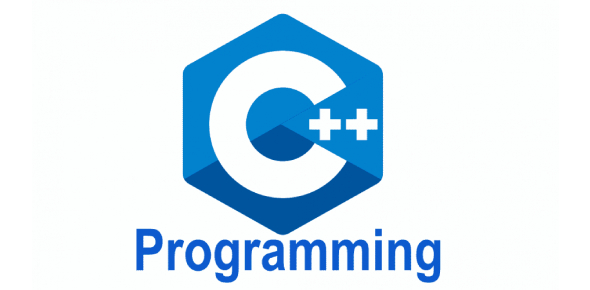# C++ Programming Trivia Questions Test! Hardest Quiz

17 Questions | Total Attempts: 695SettingsC++ Programming is one of the most common programming languages that a programmer is expected to understand in detail. Do you think you need a way of reviewing what you know about this language and its uses? The quiz below is considered the hardest, and if you can tackle it with ease, you need not worry about an exam. Try it out!

• 1.
A switch statement variable must be ________
• A.

Integer

• B.

Bool

• C.

Char

• D.

Enumerated type

• E.

All choices

• 2.
Which boolean operation is described by the following table? A           B            Operation True      True           True True      False          False False     True           False                                                                                                                                                                                     False     False          False
• A.

Or

• B.

And

• C.

Not

• D.

None are correct

• 3.
Which of the following symbols has the highest precedence?
• A.

+

• B.

||

• C.

&&

• D.

-

• 4.
If a programming language does not use short-circuit evaluation, what is the output of the following code fragment if the value of myInt is 0? int other=3, myInt; if (myInt !=0 && other % myInt !=0)      cout << "other is odd\n"; else      cout << "other is even\n";
• A.

Other is even

• B.

Other is odd

• C.

0

• D.

Run-time error, no output

• 5.
Which of the following boolean expressions tests to see if x is between 2 and 15 (including 2 and 15)?
• A.

(x = 2)

• B.

(2

• C.

(x >= 2 && x

• D.

(2

• 6.
Given the following enumerated data type definition, what is the value of SAT?          enum myType{SUN,MON,TUE,WED,THUR,FRI,SAT,NumDays};
• A.

7

• B.

6

• C.

8

• D.

5

• E.

Unknown

• 7.
What is the output of the following code fragment if x is 15?      if(x < 20)           if(x <10)                 cout << "less than 10 ";      else            cout << "large\n";
• A.

Less than 10

• B.

Nothing

• C.

Large

• D.

No output, syntax error

• 8.
What is wrong with the following switch statement? int ans; cout <<"Type y for yes on n for no\n"; cin >> ans; switch (ans) {      case 'y':      case 'Y': cout << "You said yes\n"; break;      case 'n':      case 'N': cout << "You said no\n"; break;      default: cout <<"invalid answer\n"; }
• A.

Ans is an int

• B.

Break; is illegal syntax

• C.

Nothing

• D.

There are no break statements on 2 cases.

• 9.
What is the output of the following code fragment? {      int x=13;      cout << x <<","; } cout << x << endl;
• A.

13,13

• B.

0,13

• C.

13,0

• D.

Nothing, there is a syntax error.

• 10.
Given the following code, what is the final value of i? int i; for(i = 0; i <= 4; i++) {      cout << i << endl; }
• A.

3

• B.

4

• C.

5

• D.

0

• 11.
Given the following code, what is the final value of i? int i,j; for(i=0;i<4;i++) {      for(j=0;j<3;j++)      {           if(i==2)                 break;       } }
• A.

3

• B.

4

• C.

5

• D.

0

• 12.
Which of the following is not a good reason for choosing a certain loop control?
• A.

What the loop does

• B.

The minimum number of iterations of the loop

• C.

The condition for ending the loop

• D.

If the loop is in a function

• 13.
If you need to write a do-while loop that will ask the user to enter a number between 2 and 5 inclusive, and will keep asking until the user enters a correct number, what is the loop condition?
• A.

(2

• B.

(2

• C.

(2

• D.

(2 < number || number > 5)

• E.

(2 > number && number > 5)

• 14.
Which of the following data types may be used in a switch statement?
• A.

Int

• B.

Char

• C.

Enum

• D.

Long

• E.

Int, char, enum and long

• F.

Int and long only

• 15.
Which of the following are valid case statements in a switch?
• A.

Case 1:

• B.

Case x

• C.

Case 'ab':

• D.

Case 1.5:

• 16.
What is the output of the following code fragment if x is 15?      if(x < 20)           if(x <10)                 cout << "less than 10 ";      else            cout << "large\n";
• A.

Less than 10

• B.

Nothing

• C.

Large

• D.

No output, syntax error

Related TopicsBack to top